##### Actions

(diff) ← Older revision | Latest revision (diff) | Newer revision → (diff)

An arithmetic function of one argument that satisfies the following conditions for two relatively prime integers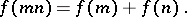An additive arithmetic function is said to be strongly additive if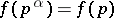for all prime numbersand all positive integers. An additive arithmetic function is said to be completely additive if the condition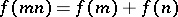is satisfied for relatively non-prime integers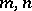as well; in such a case.

Examples. The function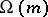, which is the number of all prime divisors of the number(multiple divisors are counted according to their multiplicity), is an additive arithmetic function; the function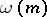, which is the number of different prime divisors of the number, is strongly additive; and the functionis completely additive.